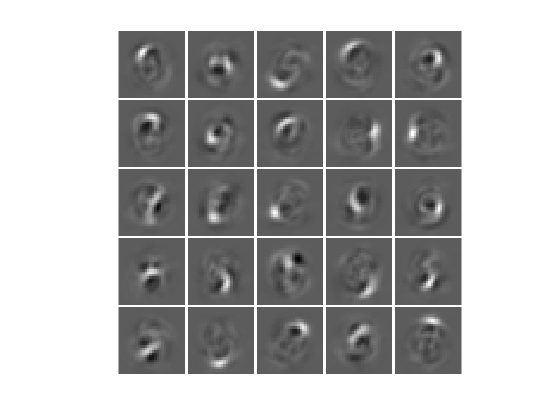Main Content

# plotWeights

Class: Autoencoder

Plot a visualization of the weights for the encoder of an autoencoder

## Syntax

``plotWeights(autoenc)``
``h = plotWeights(autoenc)``

## Description

example

````plotWeights(autoenc)` visualizes the weights for the autoencoder, `autoenc`.```
````h = plotWeights(autoenc)` returns a function handle `h`, for the visualization of the encoder weights for the autoencoder, `autoenc`.```

## Input Arguments

expand all

Trained autoencoder, returned as an object of the `Autoencoder` class.

## Output Arguments

expand all

Image object, returned as a handle.

## Examples

expand all

Load the training data.

```X = digitTrainCellArrayData; ```

The training data is a 1-by-5000 cell array, where each cell contains a 28-by-28 matrix representing a synthetic image of a handwritten digit.

Train an autoencoder with a hidden layer of 25 neurons.

```hiddenSize = 25; autoenc = trainAutoencoder(X,hiddenSize, ... 'L2WeightRegularization',0.004, ... 'SparsityRegularization',4, ... 'SparsityProportion',0.2); ```

Visualize the learned features.

```plotWeights(autoenc); ```## Tips

• `plotWeights` allows the visualization of the features that the autoencoder learns. Use it when the autoencoder is trained on image data. The visualization of the weights has the same dimensions as the images used for training.

## See Also

Introduced in R2015b

Download ebook